###### KCSE CHEMISTRY PAPER 1 2020

(THEORY)

PAPER 1

KCSE chemistry PAPER 1 2020

INSTRUCTION TO CANDIDATES

• a) Write your name and index number in the spaces provided above.
• (b) Sign and write the date of examination in the spaces provided above.

• (c) Answer all the questions in the spaces provided in the question paper.

• (d) Non-programmable silent electronic calculators may be used

• (e) This paper consists of 12 printed pages. Candidates should check the question paper to ascertain that all the pages are printed as indicated and that no questions are missing.

• ( (g) Candidates should answer the questions in English.

Q1. KCSE 2020 CHEMISTRY PAPER 1

KCSE 2020 PP1 MARKING SCHEME.

1. Element A has mass number 40 and 21 neutrons

(a) Write the electron arrangement of element

• No. of electrons 40-21=19
• Electron arrangement 2.8.8.1

(b)Give the formula of the compound formed when element A react with sulphur.(S=16.0)

• A2S or SA2  OR K2S or SK2

2.Study the set-up in Figure 1 and then answer the question that follows.(a) At the end of the experiment solid B changed from white to blue. Explain.

• Solid B become hydrated hence turning from white to blue OR
• A burning candle produces water which combines with solid B to make it hydrated.

(b) The other product of the burning candle formed a white precipitate with the Calcium hydroxide solution. Write an equation for the reaction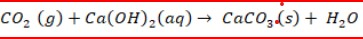(c) State the role of apparatus C.

• To suck/pull/remove the gaseous product from the system.

3. a) State and explain the factor that is considered when collecting a gas by displacement of;

(i) Air

• The density of the gas; If the gas is lighter than air- upward delivery or downward displacement is used. OR If the gas is denser than air – downwards delivery is used.

(ii) Water

• Solubility in water
• If the gas is insoluble or slightly soluble in water.

(b) Other than collecting a gas by displacement of air or water, state another method that can be used to collect a gas.

• Gas syringe
• Solidification
• Condensation
• Freezing
• Collection over mercury
• Liquidification

4. (a) Carbon (II) Oxide was passed over 4.1g of heated oxide of copper in a combustion tube until there was no further change. The mass of the final substance was found to be 3.29g .

Complete table 1 and determine the empirical formula of the oxide.

(Cu= 64.0 : O=16.0)

Table 1

 Element Cu O Mass(g) 3.29 0.81 Number of moles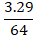= 0.051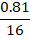= 0.051
• Mole ratio  IS 1:1
• The empirical formula is CuO

b) State the property of carbon(II) oxide that was demonstrated in the experiment.

• Reducing agent/ reducing property

5. a) Draw the structural formula of 2-methylbut-2-ene.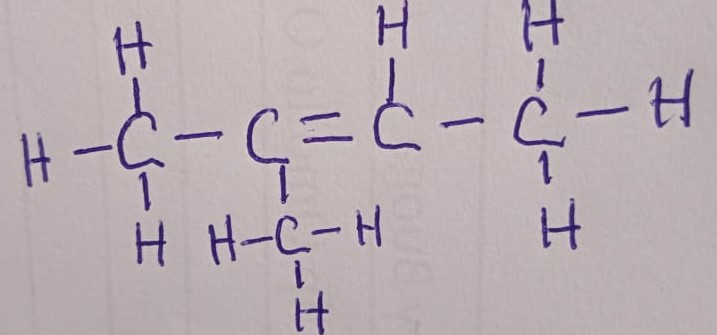(b) Bromine water was added to 2-methylbut-2-ene.

• Bromine water is decolourised
• Orange/brown/yellow bromine turns to colourless

(ii) Name the type of reaction that took place.

6. Table 2 shows pH values of solutions of compounds D, E, F and G.

Table 2

 Compound D E F G pH value of the solution 2 5 7 13

(a) State which one of the compounds is likely to be;

(i) Sodium chloride;

• F of pH 7

(ii) Ammonium nitrate;

• E of pH 5

(b) Select two compounds that can be used to illustrate the amphoteric nature of an oxide.

• D and G OR 2 and 13

(c) Give a reason for the answer in (b)

• Amphoteric oxide reacts with both strong acids /acids and alkalis OR
• Amphoteric oxides behave as acids or bases

7. Draw a labelled diagram of the setup of apparatus that can be used to electrolyse lead(II) bromide.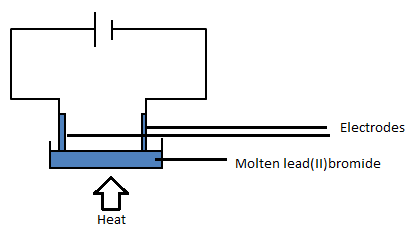8. a) State the difference between a covalent bond and a dative bond.

• In a covalent bond, the shared electrons are contributed by both the atoms  WHILE In a dative bond the shared electrons are donated by one atom.

b) Using dots(.) and crosses (×) to represent electrons, draw a diagram to show the bonding in ammonia.

c) Using the diagram in (b), state one property that makes ammonia react with hydrogen ions.

• Nitrogen in ammonia contains one pair of electrons OR
• Presence of one pair of electron/unshared pair of electrons

9. Figure 2 shows a reaction scheme starting with copper turnings. Study it and answer the questions that follow.

(a) State the reagent that is added in step 1.

• Concentrated sulphuric (VI) acid or conc. H2S04  OR
• Sulphuric (VI) acid.

(b) Identify gas H.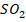OR   Sulphur (IV) oxide

(c) Write the formula of the complex ion in solution J.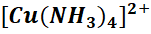10. When chlorine is bubbled through hot concentrated sodium hydroxide solution, sodium chlorate (V), sodium chloride and water are formed.

(a)  Write the equation for the reaction.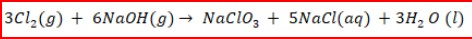(b) Sodium chlorate (V) and sodium chloride have different solubilities in water. Name a method that can be used to separate the salts.

• Fractional crystallization.

(c) Give one use of sodium chlorate (V).

• Herbicides
• Bleaching agent
• Treatment of sewage
• Manufacture of dyes
• In fireworks and explosive machines

11. Excess dilute hydrochloric acid was added to an alloy of copper and zinc in a beaker.

• Fizzing/Effervescence/Production of gas bubbles
• Colourless solution formed
• Brown residue/solid

(b) Excess aqueous sodium hydroxide was added to 2 cm3 of the solution obtained in the reaction. Write an equation for the reaction that occurred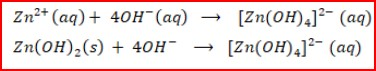12. Study the information in Table 3 and answer the questions that follow. The elements belong to the same chemical family. (The letters are not actual symbols of the elements).(a) Classify the elements as either metals or non-metals. Give a reason.

(b) i.  Identify the elements which are;

I]  Least reactive:         M

II]  Most reactive:           N

ii.   Give a reason for the answer in b (i)

• The more the ionization energy the less the reactive OR
• The less the ionization energy the more the reactive/ can also use atomic radius to explain.

13. Nitrogen (IV) Oxide is prepared by heating lead(II) nitrate.

(a) Write an equation for the reaction.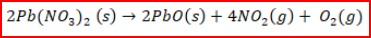(b) At room temperature, nitrogen (IV) oxide exists as an equilibrium mixture with dinitrogen tetraoxide.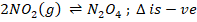(brown)           (pale yellow)

State the observation made when the mixture is placed in an ice bath. Give a reason.

• The mixture turns yellow/ yellow colour intensifies
• A decrease in temperature forward is favoured/ more N2O4  is formed since the reaction is exothermic.

14. Figure 3 shows an energy level diagram for the decomposition of hydrogen peroxide using a catalyst.

1. Using the energy values A, B and C , write an expression for:
1. ∆H of the reaction;
• ∆H = A-B
1. Activation energy;
• EA =C – B
1. On the same axis, sketch a curve that would be obtained if the reaction was carried out without a catalyst.

15. Sodium carbonate is prepared on a large scale by the  Solvay process. The equation for the main reaction that takes place in the carbonator is:

NaCl (aq)